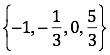# Keeping in Line

## OBJECTIVE: To learn how to graph points on the real number line

In a previous lesson, you learned about the set of real numbers and its subsets. Keeping It Real: https://www.geogebra.org/m/n8hauvp6 In this lesson, you'll learn how to construct the real number line and plot points on it. Below is a real number line with the graph of a few points. Let's first examine the parts. Real Number Line—line where numbers are associated with points Origin—arbitrary point labeled as zero (0) Graph—point associated with a number (in the real number line below, X and Y are points) Coordinate—number associated with a point (in the real number line below, -1 and 3 are coordinates)

## HOW TO CONSTRUCT THE REAL NUMBER LINE

Step 1. ﻿Draw a horizontal line with arrowheads on both ends. Choose any point to represent zero (0); this point is the origin.
Step 2. ﻿Mark off consistent lengths on both sides of the origin and label each tick mark using evenly-spaced numbers (e.g., integers, even numbers, multiples of 5, etc.) to define the scale.
Step 3. ﻿The graph of each real number is shown as a dot at the appropriate point on the number line. The graphs of the integers from -6 to 6 are shown below.

## Example:

﻿ Graph the following set of real numbers:## TRY IT YOURSELF ...

Use the applet below to help you locate and place numbers on a number line. Click on the "New Target Number" button, and move the point along the number line to where you think the point in APPROXIMATELY located. Click on the "Check Answer" button. If you need to try again, click on the "Check Answer" button again to keep trying! You may pan and zoom the line.

## In this lesson, you learned how to construct the real number line and plot points on it.

In future lessons, you'll learn more about the real number line and graphing subsets of real numbers on it. Did you ENJOY today's lesson?